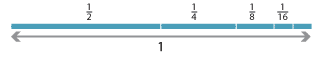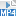## Content

### Limiting sums

A full study of infinite series is beyond the scope of the secondary school curriculum. But one infinite series, which was studied in antiquity, is of particular importance here.

Suppose we take a unit length and divide it into two equal pieces. Now repeat the process on the second of the two pieces, and continue in this way as long as you like.Dividing a unit length into smaller and smaller pieces.

This generates the sequence

$\dfrac{1}{2}, \dfrac{1}{4}, \dfrac{1}{8}, \dfrac{1}{16}, \dots.$

Intuitively, the sum of all these pieces should be 1.

After $$n$$ steps, the distance from 1 is $$\dfrac{1}{2^n}$$. This can be written as

$\dfrac{1}{2} + \dfrac{1}{4} + \dfrac{1}{8} + \dots + \dfrac{1}{2^n} = 1 - \dfrac{1}{2^n}.$

The value of the sum approaches 1 as $$n$$ becomes larger and larger. We can write this as

$\dfrac{1}{2} + \dfrac{1}{4} + \dfrac{1}{8} + \dots + \dfrac{1}{2^n} \to 1 \quad \text{as} \quad n \to \infty.$

We also write this as

$\dfrac{1}{2} + \dfrac{1}{4} + \dfrac{1}{8} + \dots = 1.$

This is an example of an infinite geometric series.

A series is simply the sum of the terms in a sequence. A geometric sequence is one in which each term is a constant multiple of the previous one, and the sum of such a sequence is called a geometric series. In the example considered above, each term is $$\dfrac{1}{2}$$ times the previous term.

A typical geometric sequence has the form

$a, ar, ar^2, ar^3, \dots, ar^{n-1}$

where $$r \neq 0$$. Here $$a$$ is the first term, $$r$$ is the constant multiplier (often called the common ratio) and $$n$$ is the number of terms.

The terms in a geometric sequence can be added to produce a geometric series:

$S_n = a + ar + ar^2 + ar^3 + \dots + ar^{n-1}. \qquad\qquad (1)$

We can easily find a simple formula for $$S_n$$. First multiply equation (1) by $$r$$ to obtain

$rS_n = ar + ar^2 + ar^3+ \dots + ar^{n}. \qquad\qquad (2)$

Subtracting equation (2) from equation (1) gives

$S_n - rS_n = a - ar^n$ from which we have $S_n = \dfrac{a(1-r^n)}{1-r}, \qquad \text{for } r \neq 1.$

Now, if the common ratio $$r$$ is less than 1 in magnitude, the term $$r^n$$ will become very small as $$n$$ becomes very large. This produces a limiting sum, sometimes written as $$S_\infty$$. Thus, if $$|r|<1$$,

\begin{align*} S_\infty &= \lim_{n\to \infty} S_n\\ &= \lim_{n\to \infty} \dfrac{a(1-r^n)}{1-r} = \dfrac{a}{1-r}. \end{align*}

In the example considered at the start of this section, we have $$a=\dfrac{1}{2}$$ and $$r=\dfrac{1}{2}$$, hence the value of the limiting sum is $$\dfrac{\dfrac{1}{2}}{1-\dfrac{1}{2}} = 1$$, as expected.

Exercise 2

Find the limiting sum for the geometric series

$\dfrac{3}{2} + \dfrac{9}{8} + \dfrac{27}{32} + \dotsb.$

Screencast of exercise 2Next page - Content - Limit of a function at infinity